Courses

# Logical Effort Electrical Engineering (EE) Notes | EduRev

## Electrical Engineering (EE) : Logical Effort Electrical Engineering (EE) Notes | EduRev

The document Logical Effort Electrical Engineering (EE) Notes | EduRev is a part of Electrical Engineering (EE) category.
All you need of Electrical Engineering (EE) at this link: Electrical Engineering (EE)

Objectives

In this lecture you will learn the following

• Motivation for Logical Effort
• Definition of Logical Effort
• Delay in a Logical Gate

21.1 Motivation for Logical Effort

Here, we are going to introduce the concepts on which logical effort is based. Logical effort as introduced by Sutherland et al is just a formalized representation of these concepts. The propagation delay of a MOS transistor depends on the capacitance of the transistor. So, as the width W is increased capacitance increases and so does the propagation delay. Let us say that when Cin, the input capacitance of a gate (say inverter) is equal to CL, the load capacitance, then the propagation delay of the gate is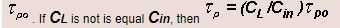Now let us see that if we can introduce a buffer then can we reduce the propagation delay of the gate (see figure 21.11).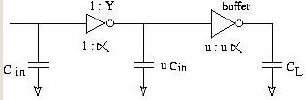Fig 21.11: A circuit for propagation delay calculation

Here, the input capacitance of 1st gate is Cin and the load capacitance is Cl. The input capacitance of 2nd gate, which is also load to the 1st, is

Now,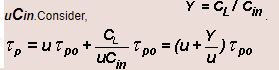if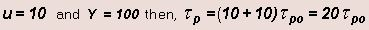Now, let us find out the optimum CL for which introducing a buffer will provide Cin performance improvement. For this we use the following: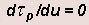Solving this differential equation leads to the following result,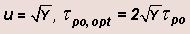From the above result, we observe that a buffer will yield better results for Y > 4. Here the input capacitance of first gate is Cin,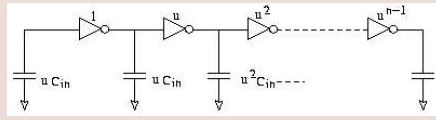Fig 21.12: A circuit of high order for propagation delay calculation

that of 2nd is uCin, hat of next is uand so on and for the last one the input capacitance is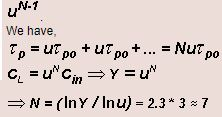taking lnu=1 and Y=1000. Therefore, with 7 stages we can drive a load capacitance 1000 times theinput capacitance.

Such cases arise when we have situations where we have to drive, lets say, a motor. Then Y> 1000 and large currents have to be delivered. In such cases where load current is to be provided outside chip then buffer should be put very close to the output pad to avoid adding line capacitance.

21.2 Definition of Logical Effort

The method of logical effort is an easy way to estimate the delay in an MOS circuit. The method can be used to decide the number of logic stages on a path and also what should be the size of the transistors. Using this method we can do a simple estimations in the early stages of design, which can be a starting point for more optimizations.

The logical effort of a gate tells how much worse it is at producing output current than an inverter, given that each of its inputs may contain only the same input capacitance as the inverter. Reduced output current means slower operation, and thus logical effort number for a logic gate tells how much more slowly it will drive a load than an inverter would.

Equivalently, logical effort is how much more input capacitance a gate presents to deliver the same output current as an inverter.

As we can see from the table presented above, the logical effort increases as the complexity of a gate increases. Also, for the same logic gate, as the number of inputs increases, the logical effort increases. Thus, larger or more complex logic gates will
exhibit more delay.

Thus we can evaluate different choices of logical structure by considering their logical effort. For example, designs that minimize the number of stages will require more inputs for each logic gate and thus have larger logical effort. Similarly, designs with fewer inputs and thus less logical effort per stage may require more stages of logic. These tradeoffs should be evaluated for an optimum design.

21.1 Delay in a Logic Gate

Delays in a MOS gate are caused by the capacitive loads and due to the gate topology. We will take an inverter as the unit gate and compare performance of other gates with an inverter. A complex logic gate, which may have transistors connected in series, will have more delay than an inverter with similar transistor sizes that drives the same load, as they are poorer at driving current. The method of logical effort quantifies these effects.

We will consider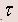as the delay unit that characterizes a given MOS process.is about 50ps for a typical 0.6 μ process.

The absolute delay of the gate is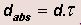, Where d is unitless delay of the gate

The delay incurred by a logic gate can be expressed as, d = f + p , Where p is a fixed part called parasitic delay and

f is proportional to the load on the gate’s output called the effort delay or stage effort. d is measured in units of.
The effort delay f depends on the load and on the properties of the logic gate driving that load and comprises of two components. f = gh ,Where g, logical effort, accounts for the properties of the gate h, electrical effort, characterizes the load.

Combining above equations, we get - d = gh + p

Thus, we see that there are four components that basically contribute to delay, namely,, g, h and p. The process parameterrepresents the speed of the basic transistor. The parasitic delay, p, represents the intrinsic delay of the gate due to its own internal capacitance. The electrical effort, h, is Cout/Cin, where Cout is the capacitance due to the load and Cin is the capacitance due to sizes of the transistors. The logical effort, g, expresses the effect of circuit topology and is independent of load and transistor sizing. Thus logical effort depends only on circuit topology.

Offer running on EduRev: Apply code STAYHOME200 to get INR 200 off on our premium plan EduRev Infinity!

,

,

,

,

,

,

,

,

,

,

,

,

,

,

,

,

,

,

,

,

,

;